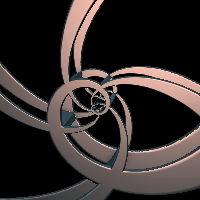# spiralZoom

Keywords:
• log
• polar
• spiral
filter
spiralZoom
RayTK
v0.36

Transforms space using a logarithmic spiral.

In a sense, this has some similar properties to `modulo2D` in that it takes a slice of space and repeats it. But instead of repeating it linearly along the x and y axes, it does so with polar coordinates (angle and distance).

Important note: if the input pattern / shape does not tile correctly for the slice that’s used, you will get a discontinuity (break in space). This is similar to how you shapes can get cut off when using `modulo1D` / etc.

When using 2D coordinates, the `Axis` is ignored, and the first axis is always X and the second is always Y.

## Parameters

• `Enable`:
• `Axis`: The axis around which to spiral. The position on this axis will stay the same. The position on the other two axes will be wrapped around this axis.
• `X`: Spiral the Y and Z axes around the X axis. First axis: Y, second: Z, around: X.
• `Y`: Spiral the Z and X axes around the Y axis. First axis: Z, second: X, around: Y.
• `Z`: Spiral the X and Y axes around the Z axis. First axis: X, second: Y, around: Z.
• `Center`: The center position along the two spiralled axes. Note that the parts of this will control different axes on the selected `Axis`.
• `Twist 1`: The amount of twisting to apply to the first axis.
• `Twist 2`: The amount of twisting to apply to the second axis.
• `Phase`: Shifts coordinates along the first and second axes, which has the effect of "spinning" different parts of the pattern.
• `Branches`: How many "arms" or "branches" of the spiral there should be. This is controls how many times the first axis repeats as it goes around the axis. Note that if this is not a whole integer, there will be a break in the spiral.

## Inputs

• `definition_in`: (required)
• Coordinate Types: `vec2` `vec3`
• Context Types: `Context` `MaterialContext` `CameraContext` `LightContext` `RayContext` `ParticleContext`
• Return Types: `float` `vec4` `Sdf` `Ray` `Light` `Particle`
• `Twist Field`: (optional)
• Coordinate Types: `vec2` `vec3`
• Context Types: `Context` `MaterialContext` `CameraContext` `LightContext` `RayContext` `ParticleContext`
• Return Types: `vec4`
• `Phase Field`: (optional)
• Coordinate Types: `vec2` `vec3`
• Context Types: `Context` `MaterialContext` `CameraContext` `LightContext` `RayContext` `ParticleContext`
• Return Types: `vec4`

## Variables

• `logdist`:
• `dist`: